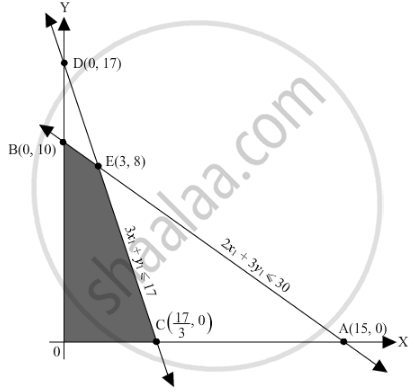# A Producer Has 30 and 17 Units of Labour and Capital Respectively Which He Can Use to Produce Two Type of Goods X and Y. - Mathematics

Sum

A producer has 30 and 17 units of labour and capital respectively which he can use to produce two type of goods x and y. To produce one unit of x, 2 units of labour and 3 units of capital are required. Similarly, 3 units of labour and 1 unit of capital is required to produce one unit of y. If x and y are priced at Rs 100 and Rs 120 per unit respectively, how should be producer use his resources to maximize the total revenue? Solve the problem graphically.

#### Solution

Let $x_1$ and $y_1$ units of goods x and y were produced respectively.
Number of units of goods cannot be negative.
Therefore,$x_1 , y_1 \geq 0$
To produce one unit of x, 2 units of labour and for one unit of y, 3 units of labour are required $2 x_1 + 3 y_1 \leq 30$
To produce one unit of x, 3 units of capital is required and 1 unit of capital is required to produce one unit of

$3 x_1 + y_1 \leq 17$

If x and y are priced at Rs 100 and Rs 120 per unit respectively, Therefore, cost of x1 and y1 units of goods x and y is Rs 100x1 and Rs 120y1 respectively.
Total revenue = Z = $100 x_1 + 120 y_1$ which is to be maximised.

Thus, the mathematical formulat​ion of the given linear programmimg problem is
Max Z =  $100 x_1 + 120 y_1$
subject to
$2 x_1 + 3 y_1 \leq 30$
$3 x_1 + y_1 \leq 17$
$x, y \geq 0$

First we will convert inequations into equations as follows:
2x1 + 3y1 = 30, 3x1 + y1 = 17, x = 0 and y = 0

Region represented by 2x1 + 3y1 ≤ 30:
The line 2x1 + 3y1 = 30 meets the coordinate axes at A(15, 0) and B(0, 10) respectively. By joining these points we obtain the line
2x1 + 3y1 = 30. Clearly (0,0) satisfies the 2x1 + 3y1 = 30. So, the region which contains the origin represents the solution set of the inequation 2x1 + 3y1 ≤ 30.

Region represented by 3x1 + y1 ≤ 17:
The line 3x1 + y1 = 17 meets the coordinate axes at

$C\left( \frac{17}{3}, 0 \right)$ and $D\left( 0, 17 \right)$ respectively. By joining these points we obtain the line
3x1 + y1 = 17. Clearly (0,0) satisfies the inequation 3x1 + y1 ≤ 17. So,the region which contains the origin represents the solution set of the inequation 3x1 + y1 ≤ 17.

Region represented by x1 ≥ 0 and y1 ≥ 0:
Since, every point in the first quadrant satisfies these inequations. So, the first quadrant is the region represented by the inequations x ≥ 0, and y ≥ 0.
The feasible region determined by the system of constraints 2x1 + 3y1 ≤ 30, 3x1 + y1 ≤ 17, x ≥ 0 and y ≥ 0 are as follows.The corner points are B(0, 10), E(3, 8)  and  $C\left( \frac{17}{3}, 0 \right)$

The values of Z at these corner points

 orner point Z=  $100 x_1 + 120 y_1$ B 1200 E 1260 C $\frac{1700}{3}$

The maximum value of Z is 1260 which is attained at E(3, 8).
Thus, the maximum revenue is Rs 1260 obtained when 3 units of x and 8 units of y were produced.are as follows

Concept: Graphical Method of Solving Linear Programming Problems
Is there an error in this question or solution?

#### APPEARS IN

RD Sharma Class 12 Maths
Chapter 30 Linear programming
Exercise 30.4 | Q 27 | Page 54